## General Chemistry

Learn the toughest concepts covered in Chemistry with step-by-step video tutorials and practice problems by world-class tutors

22. Organic Chemistry

# Alkenes and Alkynes

The presence of pi bonds in both alkenes and alkynes allows them to undergo Addition Reactions

Halogenation
1
concept

## Determine the major product from the following Halogenation reaction2m
Play a video:
Hey, guys, In this new video, we're gonna take a look at Halabja Nation. So here we're going to say that al keens and all kinds undergo addition reactions or adding to them, in which elements add across their pie bonds to create new Sigma bonds. Remember, Pi Bon is a double bond or triple bond. Sigma bonds are just single bonds now under Halogen Nation to Hala Jin's will add toe one pie bond. So we need one mole off X two for one double bond for one pie bond. Okay, so we need to halogen. So that's X two. So one mole of X two for every pi bon and here X can either be C, l or B are those tend to be the best intelligence because again, flooring is too reactive. Aydin is too slow. So here determine the major product from the following halogen nation reaction. So here goes. Are double bond it shared by this carbon here in this carbon here now, in halogen nation, what happens here is that double bond is broken so that each carbon can receive a halogen. So doing that, this is what we would get ch three this ch would gain one of those chlorine because there's two of them. The double bond is broken, but there's still a single bomb there. And then that ch two would also receive Ah, chlorine. So we would make here is a die Khallad di Howard meeting to Hala Jin's and not just die Halid, but something called a Fastenal di Hallett Vis any ALS refers to vicinity, meaning that the two halogen are on neighboring carbons. So again, the scene you'll die. How to refers to the same vicinity. The two carbons with the halogen, our neighboring carbons that's called the Fastenal Die Hallett. So we've seen this first one. Let's see if you guys could attempt to do this next one. Now here we have a triple bond, remember, we have two pi bonds. Remember, It takes one mole of X to to remove one pi bon. And since we have two pi bonds in the triple bond, that's why we require two moles of this halogen. So do what we've done before. Get a final answer, come back and take a look at my explanation and see if your answer matches mine. Good luck, guys.

Under a Halogenation reaction 1 mole of Br2 or Cl2 is added to 1 pi bond to create a dihalide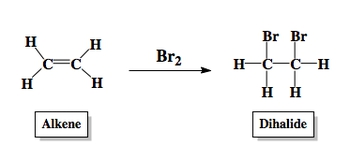2
example

## Determine the major product from the following Halogenation reaction1m
Play a video:
All right guys, let's attempt to the example that was left on the bottom of the page. So here it says, determine the major product for the following halogen nation reaction. So again, we have two pie bonds. That's why we use two moles of B R two. So this carbon and this carbon is triple bonded carbons. Each one gets two halogens apiece. Because why we have two moles of BR 2, which means we have a total of for brom means at our disposal. So two of them will go to one triple bonded carbon. The other two will go to the other triple bonded carbon. And remember when we're adding bees, these will remove the pi bonds. So we have this C H three carbon here connected to this carbon which was once triple bonded, but no longer. It has now 2 BRS on it. It's connected to the other carbon that was triple bonded at one point but no longer. And it has 2 BRS on it and then we're connected to the rest of the chain. So that's what we'd get there. We'd get four halogens being added and this would be called a tetra meaning four halid. So this would be a tetra halid. So just remember one mole of B R two or C L two helps to add two halogens onto a double bond. It removes the double bond to add two halogens. But here we have two pi bonds because it's a triple bond. So we need two moles of B R two to get our final answer.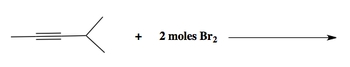Hydrogenation
3
concept

## Understanding Hydrogenation1m
Play a video:

Under Hydrogenation an alkene or alkyne reacts with hydrogen gas (H2) over a metal catalyst in order to remove pi bonds.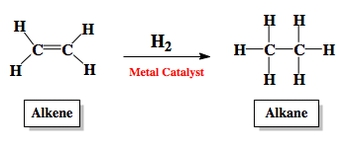4
example

## Determine the major product from the following Hydrogenation reaction1m
Play a video:
Alright, guys. So let's attempt to do that hydrogenation question that was left. So here it says, determine the major product for the following hydrogenation reaction. So we're gonna basically add Ah, hydrogen to this double bonded carbon in this double bonded carbon and adding hydrogen gets rid of that double bond. So what you're gonna get here at the end would be this structure. So you literally just take off the double bond. And remember, we don't show the hydrogen is because in a skeletal formula, we're going to say here that hydrogen is connected to carbon are invisible, so the carbons are invisible and the hydrogen is connected to them are invisible, so that will be our answer there. Now, look at the trend. We used one mole to get rid of one double bond. So knowing that, try to answer the following question where I give you a structure and I'm asking how many moles of H two would be necessary to completely reduce the structure. So just recall what one mole of h two conduce you. How many pi bonds total do we have in this molecule? That is the answer. That's the key To answer this question correctly. Good luck, guys.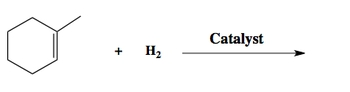5
example

## How many moles of hydrogen would be needed to completely reduce the following compound1m
Play a video:
alright, guys. So let's try to attempt to answer this question. I was left on the bottom dealing with hydrogenation. So remember, we need one mole off H two to remove one pie bond. And remember, a double bond has one pie bond and one Sigma bond. We don't care about the Sigma because we can't get rid of Sigma's. A triple bond has two PI bonds and one Sigma bond. So if we look, what do we have? We have one double bond, so that's one pie. We have another double bond, so that's a second. And then we have a triple bond, which adds another two pi bonds. So we have a total off four pi bonds. And remember, for every pi bon, we need one mole of H two. Since we have four pi bonds, that means we need four moles of H two to completely reduced this compound. So our answer here would be four moles of H two and using formals of H two on this. It would give us this as our answer. We get rid of that double bond in the ring, these two carbons, each of them would get another hydrogen the double bomb would disappear. You can draw the hydrogen anyway you want, and then here there's a carbon there. But there's also a carbon here that's not shown. So they gained two. Hydrogen is there, and that would be your answer at the end, where we've completely reduced it by adding four moles of H two.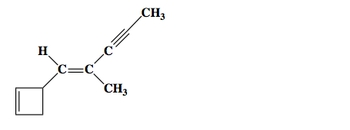Hydrohalogenation
6
concept

## Understanding Hydrohalogenation2m
Play a video:
Hey, guys, In this new video, we're gonna take a look at Hydra Halogen Nation. Now, under Hydrology Nation, a hydrogen and a halogen are added across a pi bon. So remember, hydro does not mean water. Hydro means hydrogen. So we're adding Ah, hydrogen in a halogen. Now, hear your book doesn't explicitly say this, but what we're doing here is we're following something called Markov Knockoffs rule when it comes to organic Cam, Russia and Germany and all these European countries have contributed a vast amount of knowledge in a lot of these reactions. One of the guys to help with this type of reaction was Markov Nankov. So they named it the Markov Markov's rule. Now, under my Kafelnikov's rule, you're gonna focus on the two double bonded carbons. You're going to say the hydrogen goes to the double bonded carbon with mawr. Hydrogen is on it, and then the halogen goes to the double bonded carbon with less hydrogen. So here, the re agent that we use in this reaction is HCL and HBR normally, But we could also use H F and H I HCL in hbr just happened to be the major forms that you'll see now again, I've said this multiple times. We get to organic chemistry. You gonna learn that hydrogenation is not as simple as this. And there are things called rearrangements that to occur here. Luckily, because you guys are learning the beginnings of organic chemistry, you don't have to worry about that. So just simply follow what I said here. This is just Markov. No cost rule Markov Makov Rule tells us h goes tome or hydrogen halogen goes toe less so knowing that we can use that Marco Viticultural to answer these questions. So attempt to do this one on your own Focus on the double bonded carbons which one has mawr hydrogen is directly connected to it will gain the hydrogen. The one that has less hydrogen is connected to it will gain the halogen. And when we're adding these two groups, what happens to our double bond? Knowing all of these things will give you the correct answer so attempted on your own and then come back and see how I approach the question

Under a Hydrohalogenation reaction an alkene or alkyne reacts with HCl or HBr to add a hydrogen and halogen to the pi bond.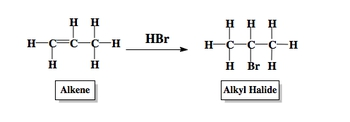7
example

## Determine the major product from the following Hydrohalogenation reaction2m
Play a video: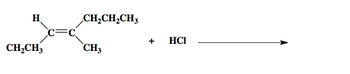8
example

## Determine the major product from the following Hydrohalogenation reaction1m
Play a video: